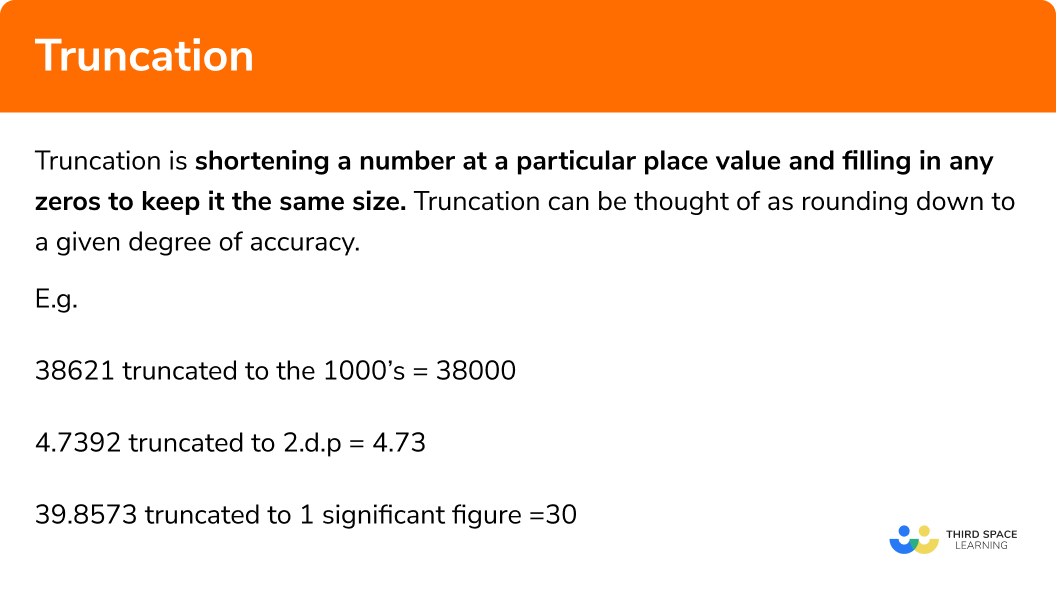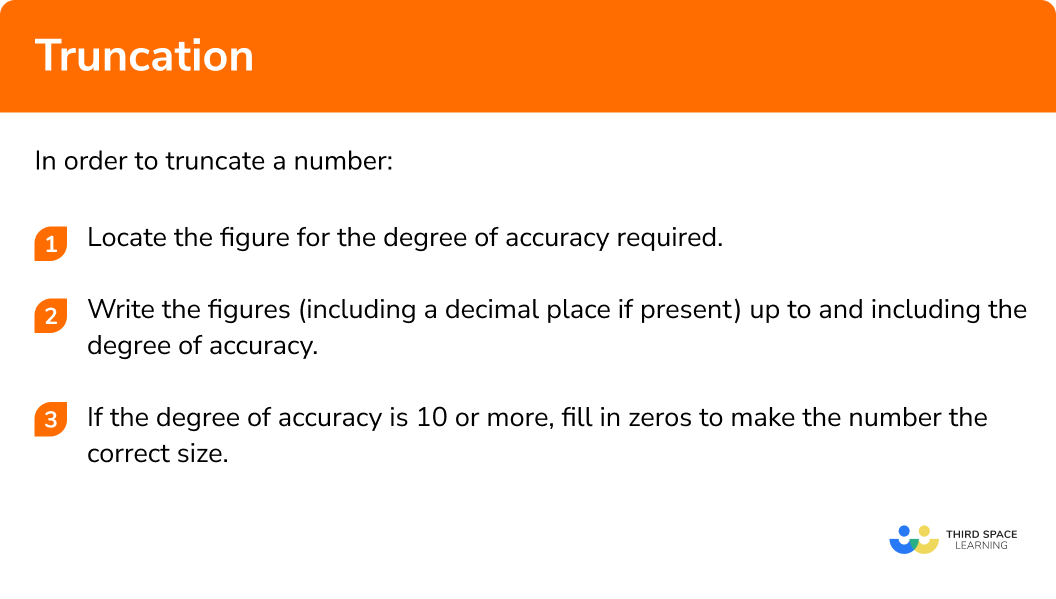# Truncation

Here we will learn about truncation including how to truncate numbers to powers of 10, decimals and significant figures.

There are also truncation worksheets based on Edexcel, AQA and OCR exam questions, along with further guidance on where to go next if you’re still stuck.

## What is truncation?

Truncation is shortening a number at a particular place value and filling in any zeros to keep it the same size.

Truncation can be thought of as rounding down to a given degree of accuracy.
To do this we write the figures of the number up to and including the place value required and then, if necessary write zeros to make the number the same size as the original number.

E.g.

38621 truncated to the 1000s

= 38000

4.7392 truncated to 2.d.p

= 4.73

39.8573 truncated to 1 significant figure

=30

### What is truncation?## How to truncate a number

In order to truncate a number:

Step 1: Locate the figure for the degree of accuracy required.

Step 2: Write the figures (including a decimal place if present) up to and including the degree of accuracy.

Step 3: If the degree of accuracy is 10 or more, fill in zeros to make the number the correct size.

### Explain how to truncate a number in 3 steps## Truncated maths examples

### Example 1: truncating to an integer

Truncate 47.893 to an integer.

1Locate the figure for the degree of accuracy required.

In 47.893, the units figure is 7

2Write the figures (including a decimal place if present) up to and including the degree of accuracy.

47

3If the degree of accuracy is 10 or more, fill in zeros to make the number the correct size.

There are no zeros required so the truncated value is 47.

### Example 2: truncating to decimal places

Truncate 2.45789 to 2 decimal places.

In 2.45789, the second decimal place is 5.

2.45

If the degree of accuracy is 10 or more, fill in zeros to make the number the correct size.

There are no zeros required so the truncated value is 2.45.

### Example 3: truncating to powers of 10

Truncate 3468 to the hundreds.

In 3468, the hundreds figure is 4

34

The degree of accuracy is 100, which has two zeros. Therefore, we need to write two zeros to make the number the correct size.

The truncated value is 3400.

### Example 4: truncating to significant figures

Truncate 26783 to one significant figure.

In 26783, the first significant figure is 2.

2

The degree of accuracy is 10000, which has four zeros. Therefore, we need to write four zeros to make the number the correct size.

The truncated value is 20000.

### Common misconceptions

• The truncated number is not the correct size

It is common for step 3 to be missed and a number such as 3547 to be truncated to 35 to 2 significant figures. It is important that the zeros are written to make the number the correct size.

• The number is rounded up instead of truncated

It is common to round up instead when the number after the figure being truncated to is 5 or more.

Truncation is part of our series of lessons to support revision on rounding numbers, and upper and lower bounds. You may find it helpful to start with the main rounding numbers lesson for a summary of what to expect, or use the step by step guides below for further detail on individual topics. Other lessons in this series include:

### Practice truncated maths questions

1. Truncate 0.0368 to 1 significant figure

30.30.030.04The first significant figure is 3. There are no zeros required so the answer is 0.03.

2. Truncate 6.256 to the units

676.26256The units figure is 6. There are no zeros required so the answer is just 6.

3. Truncate 28.985 to 1 decimal place

29.028.928.9828.99The first decimal place is 9. There are no zeros required so the answer is 28.9.

4. Truncate 21835 to the thousands

21000220002180021835The thousands figure is 1. There are three zeros required so the answer is 21000.

5. Truncate 3269 to 3 significant figures

3270326932603300The third significant figure is 6. There is one zero required so the answer is 3260.

6. Truncate 4.396836 to 3 decimal places

4.3974.3964.0004.390The third decimal place is 6. There are no zeros required so the answer is4.396.

### Truncated maths GCSE questions

1. Truncate 2.7868 to 2 decimal places

(1 mark)

 2.78

(1)

2. (a)  x is truncated to the tens and given as 400 . What the the largest possible integer value of x ?

(b)  y is truncated to the hundreds and given as 2700 . What the the largest possible integer value of y ?

(2 marks)

409

(1)

2799

(1)

3. A rectangle has a length 6.487m and a width 3.924m . By truncating the measurements to 10cm , approximate the area of the rectangle

(3 marks)

6.4  or  3.9

(1)

6.4 \times 3.9

(1)

24.96 m^2

(1)

## Learning checklist

You have now learned how to:

• Apply and interpret limits of accuracy when truncating

## Still stuck?

Prepare your KS4 students for maths GCSEs success with Third Space Learning. Weekly online one to one GCSE maths revision lessons delivered by expert maths tutors.

Find out more about our GCSE maths tuition programme.